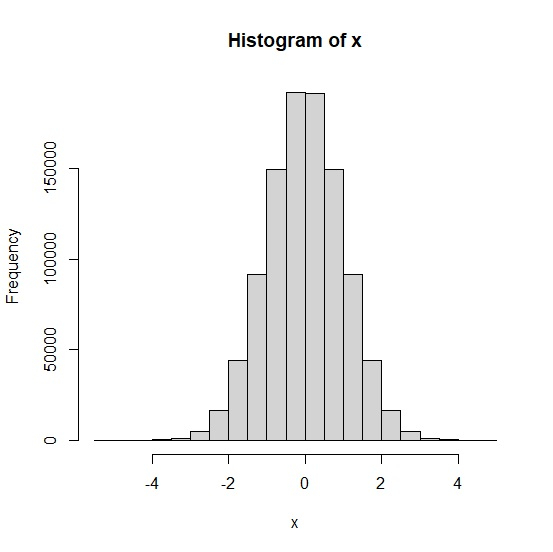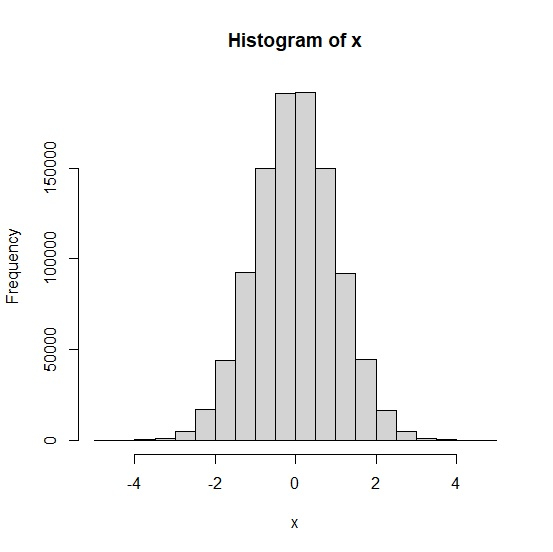# How to assign a value to a base R plot?

To assign a value to a base R plot, we can follow the below steps −

• First of all, create a vector and its histogram then record it with recordPlot function in an object.

• Then, use dev.off function to remove the plot.

• After that, read the plot with object name.

## Create the vector and histogram then save it in an object

Let’s create a vector of normal distribution and create its histogram then save it in an object called Histogram using recordPlot as shown below −

Live Demo

x<-rnorm(1000000)
hist(x)
Histogram=recordPlot()

On executing, the above script generates the below output(this output will vary on your system due to randomization) −

## Output## Remove the plot from plot window

Using dev.off function to remove the plot from plot window −

Live Demo

x<-rnorm(1000000)
hist(x)
Histogram=recordPlot()
dev.off()

## Output

null device
1

## Create the histogram again

Now we can call Histogram to create the histogram −

Live Demo

x<-rnorm(1000000)
hist(x)
Histogram=recordPlot()
Histogram

## Output